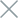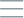• Home
• Tags
• Series
•# Lambda Functions and Functional Programming in Python

## Lambda Functions

• In Python, a `lambda` is an anonymous function created using the `lambda` keyword.
• Lambda functions consist of a single, simple expression and are often used when you need a concise function without defining a separate function.
• The syntax of a lambda function is: `lambda arguments: expression`

Example:

``````# Lambda function to calculate square
square = lambda x: x**2
print(square(5))   # Output: 25

# Lambda function to calculate the sum of two numbers
add = lambda a, b: a + b
``````

## Functional Programming

• Functional Programming is a programming style based on using functions and avoiding stateful variables.
• In Python, you can implement Functional Programming using methods like `map()`, `filter()`, `reduce()`, and lambda functions.
• These functions allow you to perform operations on data without changing their state.

Example:

``````# Using map() and lambda function to calculate squares of numbers in a list
numbers = [1, 2, 3, 4, 5]
squared_numbers = list(map(lambda x: x**2, numbers))
print(squared_numbers)   # Output: [1, 4, 9, 16, 25]

# Using filter() and lambda function to filter even numbers in a list
even_numbers = list(filter(lambda x: x % 2 == 0, numbers))
print(even_numbers)   # Output: [2, 4]
``````

Functional Programming in Python makes your code more readable, maintainable, and extensible. It also helps you avoid issues related to stateful variables and is a popular programming style in software development.